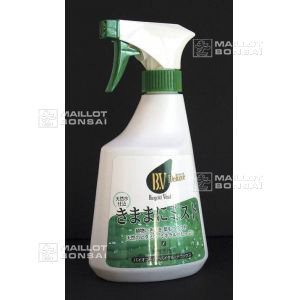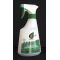Start your cart here complete your order on Maillot-Erable or Japon-Import and reduce your shipping costs##### The Japanese Bonsai specialist
Direct order Contact Help / Services Newsletter# Biogold vital de luxe bonsai spray treatment 500ml

› Bonsai soil and fertiliser › Fertilizerref. : 5223

22,00

Available quantity : 2Order

###### Description

For healthy green leaves on indoor and outdoor bonsai use this spray fertiliser to give your trees a new lease of life. Quick and easy to use, apply it to deciduous trees, conifer vegetation and kusamono small plants.

Spray bottle 500ml. Use undiluted. Ready to use.

How to use:

1. Spray on foliage 1 to 2 times per month. Rapidly absorbed. If the plant is particularly weak you can spray it once or twice every 10 days.

2. Spray on the earth once a month.

3. Soak seeds for one or two hours in the solution before sowing. This will ward off fungal attacks and aid root development.

Our range of Biogold granules complement this product.

#spray 7.5 #biogold 3.7 #bonsai 3.6 #this 3.4 #fertilizer 3 #treatment 2.9 #month 2.5 #vital 2.5 #trees 2.5 #once 2.4

Formule
(( ROUND((CHAR_LENGTH(b.article_nom)-CHAR_LENGTH(REPLACE(b.article_nom, 'spray', '')))/LENGTH('spray')) + ROUND((CHAR_LENGTH(b.article_description)-CHAR_LENGTH(REPLACE(b.article_description, 'spray', '')))/LENGTH('spray')) ) * 7.5) + (( ROUND((CHAR_LENGTH(b.article_nom)-CHAR_LENGTH(REPLACE(b.article_nom, 'biogold', '')))/LENGTH('biogold')) + ROUND((CHAR_LENGTH(b.article_description)-CHAR_LENGTH(REPLACE(b.article_description, 'biogold', '')))/LENGTH('biogold')) ) * 3.7) + (( ROUND((CHAR_LENGTH(b.article_nom)-CHAR_LENGTH(REPLACE(b.article_nom, 'bonsai', '')))/LENGTH('bonsai')) + ROUND((CHAR_LENGTH(b.article_description)-CHAR_LENGTH(REPLACE(b.article_description, 'bonsai', '')))/LENGTH('bonsai')) ) * 3.6) + (( ROUND((CHAR_LENGTH(b.article_nom)-CHAR_LENGTH(REPLACE(b.article_nom, 'this', '')))/LENGTH('this')) + ROUND((CHAR_LENGTH(b.article_description)-CHAR_LENGTH(REPLACE(b.article_description, 'this', '')))/LENGTH('this')) ) * 3.4) + (( ROUND((CHAR_LENGTH(b.article_nom)-CHAR_LENGTH(REPLACE(b.article_nom, 'treatment', '')))/LENGTH('treatment')) + ROUND((CHAR_LENGTH(b.article_description)-CHAR_LENGTH(REPLACE(b.article_description, 'treatment', '')))/LENGTH('treatment')) ) * 2.9) + (( ROUND((CHAR_LENGTH(b.article_nom)-CHAR_LENGTH(REPLACE(b.article_nom, 'trees', '')))/LENGTH('trees')) + ROUND((CHAR_LENGTH(b.article_description)-CHAR_LENGTH(REPLACE(b.article_description, 'trees', '')))/LENGTH('trees')) ) * 2.5) + (( ROUND((CHAR_LENGTH(b.article_nom)-CHAR_LENGTH(REPLACE(b.article_nom, 'month', '')))/LENGTH('month')) + ROUND((CHAR_LENGTH(b.article_description)-CHAR_LENGTH(REPLACE(b.article_description, 'month', '')))/LENGTH('month')) ) * 2.5) + (( ROUND((CHAR_LENGTH(b.article_nom)-CHAR_LENGTH(REPLACE(b.article_nom, 'vital', '')))/LENGTH('vital')) + ROUND((CHAR_LENGTH(b.article_description)-CHAR_LENGTH(REPLACE(b.article_description, 'vital', '')))/LENGTH('vital')) ) * 2.5) + (( ROUND((CHAR_LENGTH(b.article_nom)-CHAR_LENGTH(REPLACE(b.article_nom, 'once', '')))/LENGTH('once')) + ROUND((CHAR_LENGTH(b.article_description)-CHAR_LENGTH(REPLACE(b.article_description, 'once', '')))/LENGTH('once')) ) * 2.4) + (( ROUND((CHAR_LENGTH(b.article_nom)-CHAR_LENGTH(REPLACE(b.article_nom, 'luxe', '')))/LENGTH('luxe')) + ROUND((CHAR_LENGTH(b.article_description)-CHAR_LENGTH(REPLACE(b.article_description, 'luxe', '')))/LENGTH('luxe')) ) * 2.4)

## Secure payment## Delivery

Our logistic partners :## Advice, Orders

04 74 55 23 48
Pépinière MAILLOT-BONSAÏ
Le Bois Frazy
01990 RELEVANT - FRANCE
on appointment
My account My cart Newsletter RSS subscription Help / ServicesThe Japanese Bonsai specialist
If you like Maillot Bonsaï, share on  facebook

Pensez à venir nous rendre visite sur rendez vous.

Guy Maillot

OK